Скачать презентацию MSc Financial Economics The Banking System in February

db52cdb4e0c43b4162d0366c950cdd12.ppt

• Количество слайдов: 49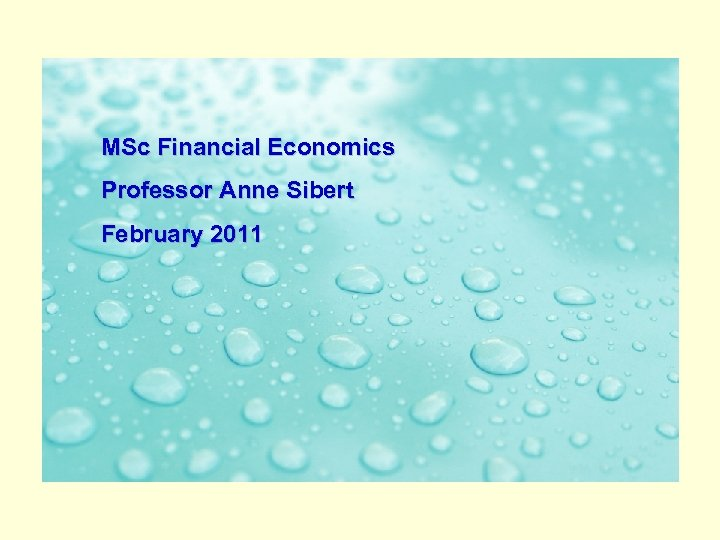MSc Financial Economics The Banking System in February 2011 an Age of Turbulence Professor Anne Sibert Professor Willem H. Buiter, London School of Economics Professor Anne Sibert, Birkbeck, University of London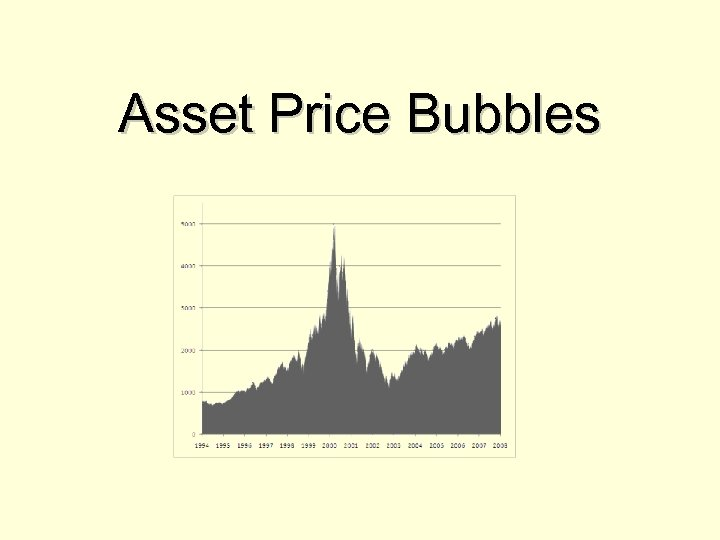Asset Price Bubbles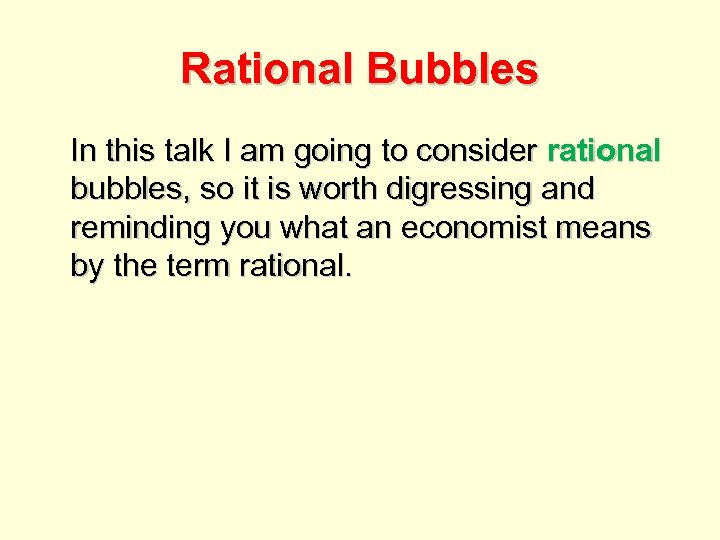Rational Bubbles In this talk I am going to consider rational bubbles, so it is worth digressing and reminding you what an economist means by the term rational.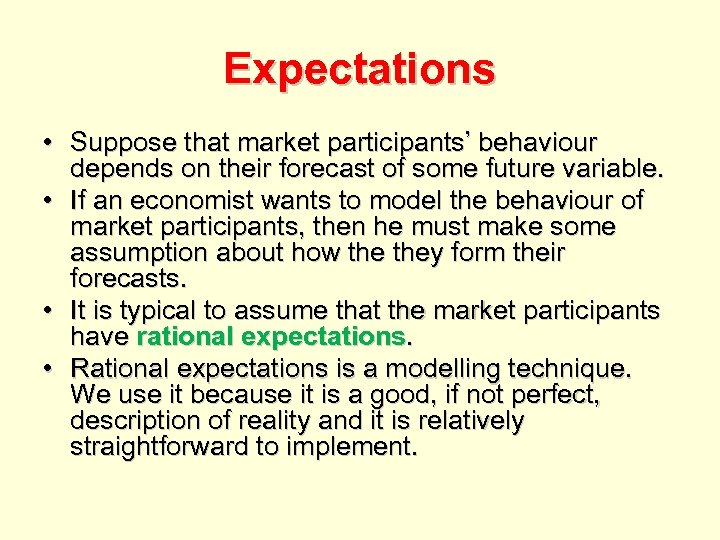Expectations • Suppose that market participants’ behaviour depends on their forecast of some future variable. • If an economist wants to model the behaviour of market participants, then he must make some assumption about how they form their forecasts. • It is typical to assume that the market participants have rational expectations. • Rational expectations is a modelling technique. We use it because it is a good, if not perfect, description of reality and it is relatively straightforward to implement.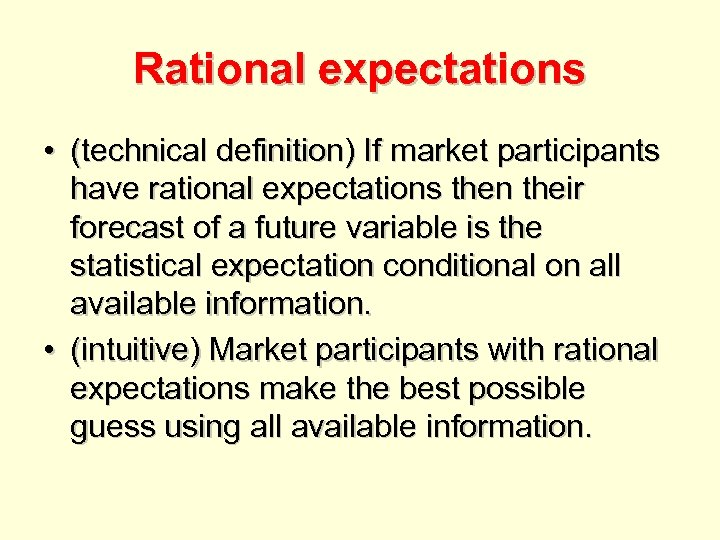Rational expectations • (technical definition) If market participants have rational expectations then their forecast of a future variable is the statistical expectation conditional on all available information. • (intuitive) Market participants with rational expectations make the best possible guess using all available information.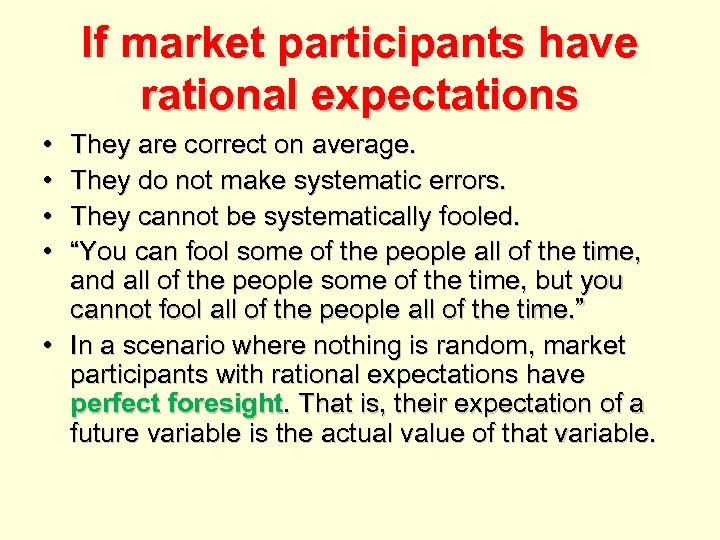If market participants have rational expectations • • They are correct on average. They do not make systematic errors. They cannot be systematically fooled. “You can fool some of the people all of the time, and all of the people some of the time, but you cannot fool all of the people all of the time. ” • In a scenario where nothing is random, market participants with rational expectations have perfect foresight. That is, their expectation of a future variable is the actual value of that variable.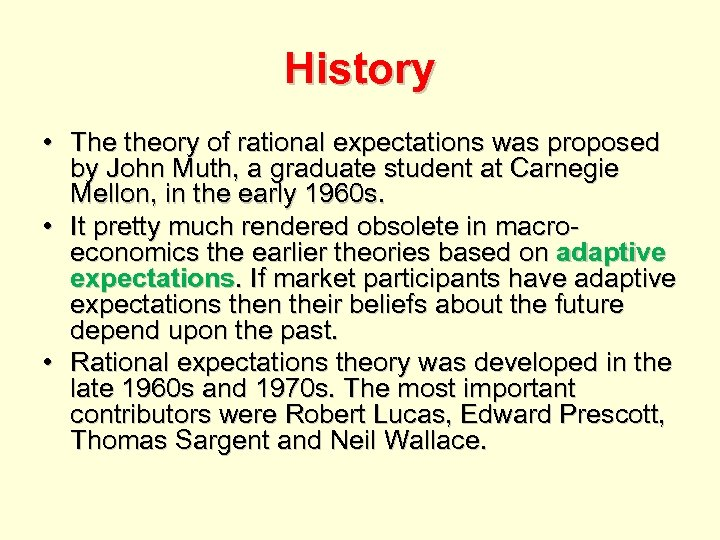History • The theory of rational expectations was proposed by John Muth, a graduate student at Carnegie Mellon, in the early 1960 s. • It pretty much rendered obsolete in macroeconomics the earlier theories based on adaptive expectations. If market participants have adaptive expectations then their beliefs about the future depend upon the past. • Rational expectations theory was developed in the late 1960 s and 1970 s. The most important contributors were Robert Lucas, Edward Prescott, Thomas Sargent and Neil Wallace.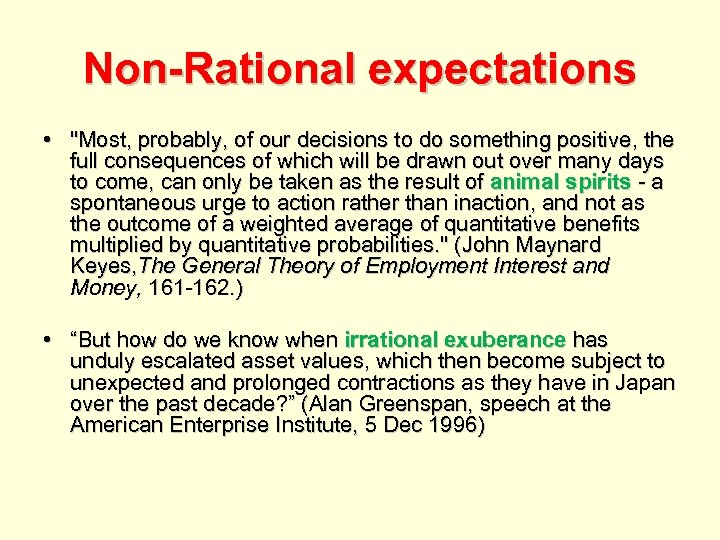Non-Rational expectations • "Most, probably, of our decisions to do something positive, the full consequences of which will be drawn out over many days to come, can only be taken as the result of animal spirits - a spontaneous urge to action rather than inaction, and not as the outcome of a weighted average of quantitative benefits multiplied by quantitative probabilities. " (John Maynard Keyes, The General Theory of Employment Interest and Money, 161 -162. ) • “But how do we know when irrational exuberance has unduly escalated asset values, which then become subject to unexpected and prolonged contractions as they have in Japan over the past decade? ” (Alan Greenspan, speech at the American Enterprise Institute, 5 Dec 1996)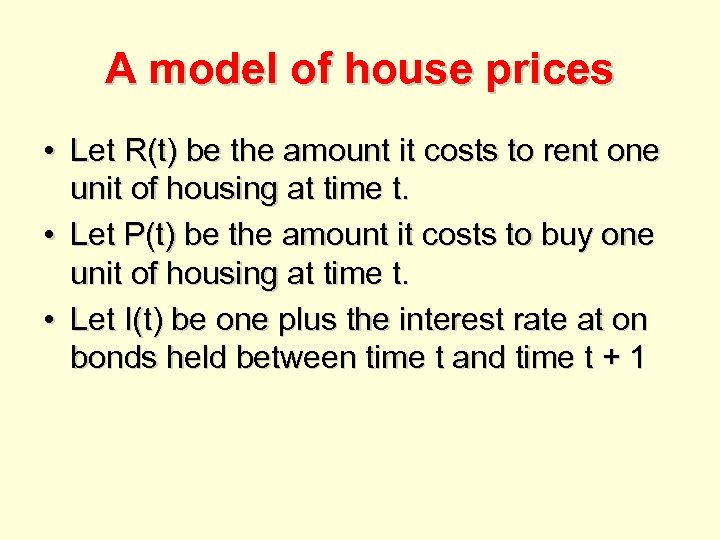A model of house prices • Let R(t) be the amount it costs to rent one unit of housing at time t. • Let P(t) be the amount it costs to buy one unit of housing at time t. • Let I(t) be one plus the interest rate at on bonds held between time t and time t + 1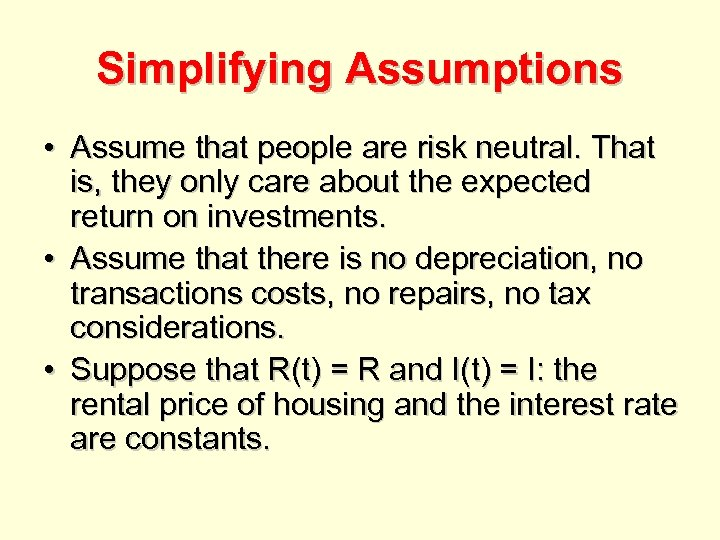Simplifying Assumptions • Assume that people are risk neutral. That is, they only care about the expected return on investments. • Assume that there is no depreciation, no transactions costs, no repairs, no tax considerations. • Suppose that R(t) = R and I(t) = I: the rental price of housing and the interest rate are constants.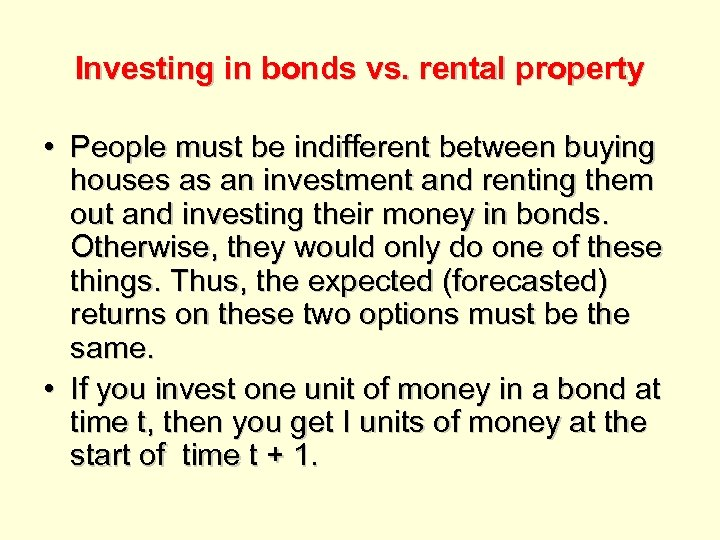Investing in bonds vs. rental property • People must be indifferent between buying houses as an investment and renting them out and investing their money in bonds. Otherwise, they would only do one of these things. Thus, the expected (forecasted) returns on these two options must be the same. • If you invest one unit of money in a bond at time t, then you get I units of money at the start of time t + 1.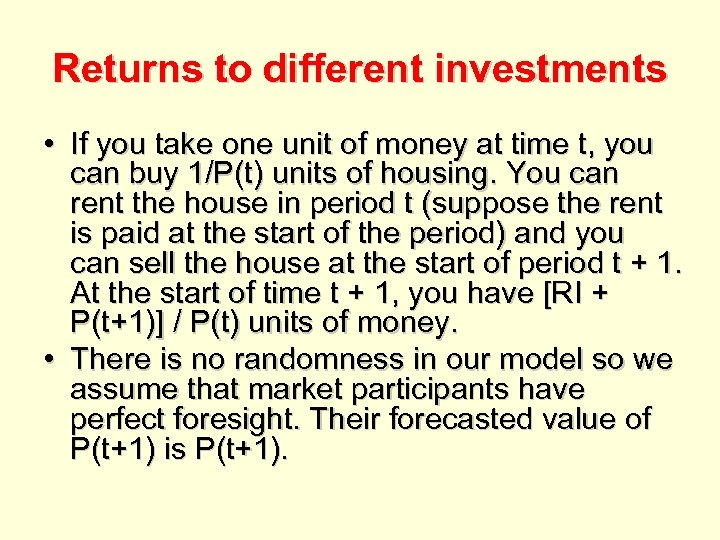Returns to different investments • If you take one unit of money at time t, you can buy 1/P(t) units of housing. You can rent the house in period t (suppose the rent is paid at the start of the period) and you can sell the house at the start of period t + 1. At the start of time t + 1, you have [RI + P(t+1)] / P(t) units of money. • There is no randomness in our model so we assume that market participants have perfect foresight. Their forecasted value of P(t+1) is P(t+1).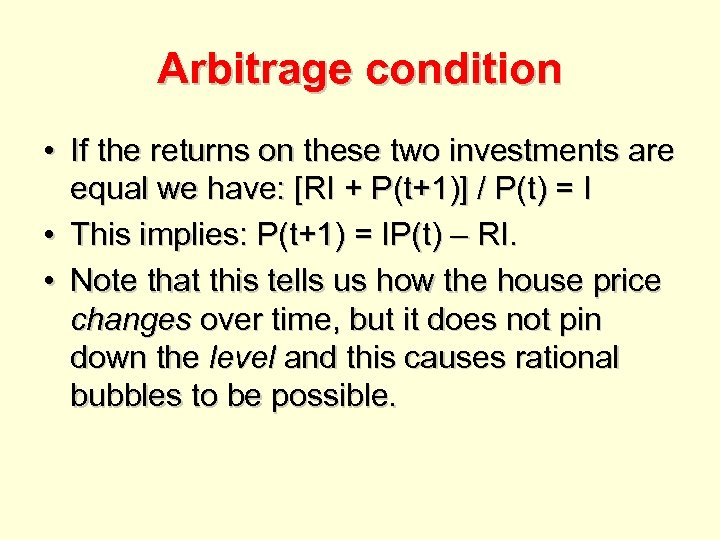Arbitrage condition • If the returns on these two investments are equal we have: [RI + P(t+1)] / P(t) = I • This implies: P(t+1) = IP(t) – RI. • Note that this tells us how the house price changes over time, but it does not pin down the level and this causes rational bubbles to be possible.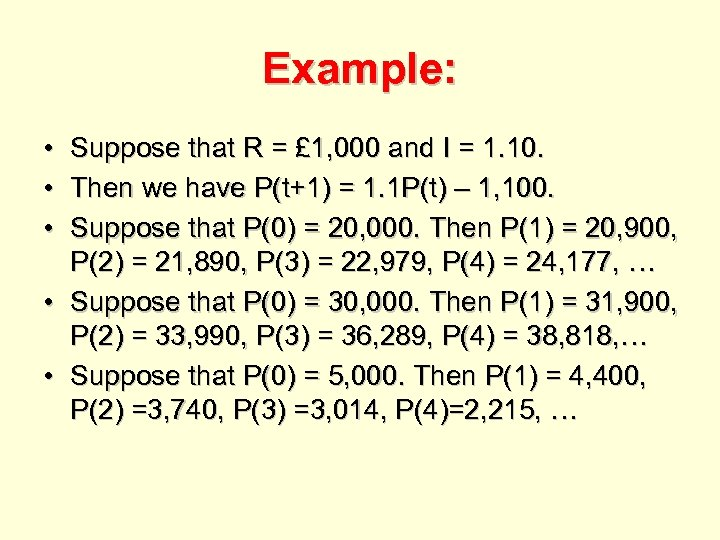Example: • Suppose that R = £ 1, 000 and I = 1. 10. • Then we have P(t+1) = 1. 1 P(t) – 1, 100. • Suppose that P(0) = 20, 000. Then P(1) = 20, 900, P(2) = 21, 890, P(3) = 22, 979, P(4) = 24, 177, … • Suppose that P(0) = 30, 000. Then P(1) = 31, 900, P(2) = 33, 990, P(3) = 36, 289, P(4) = 38, 818, … • Suppose that P(0) = 5, 000. Then P(1) = 4, 400, P(2) =3, 740, P(3) =3, 014, P(4)=2, 215, …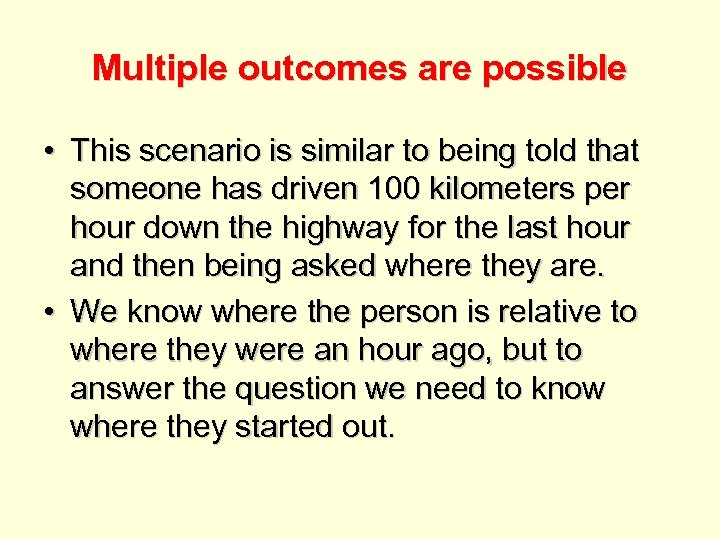Multiple outcomes are possible • This scenario is similar to being told that someone has driven 100 kilometers per hour down the highway for the last hour and then being asked where they are. • We know where the person is relative to where they were an hour ago, but to answer the question we need to know where they started out.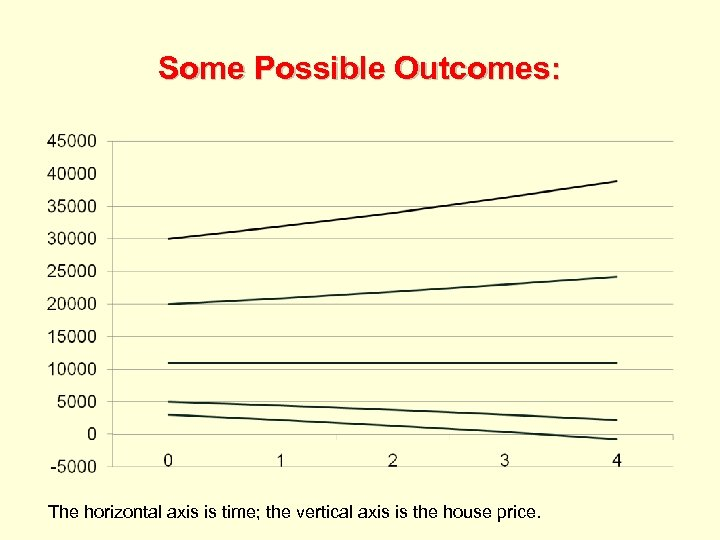Some Possible Outcomes: The horizontal axis is time; the vertical axis is the house price.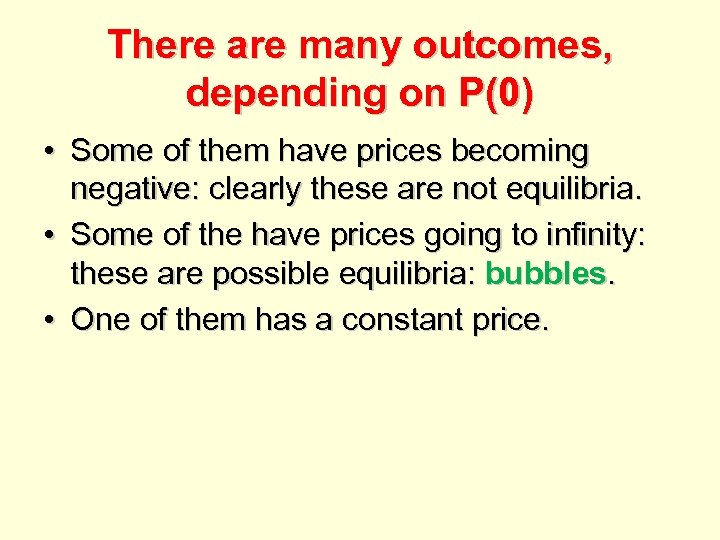There are many outcomes, depending on P(0) • Some of them have prices becoming negative: clearly these are not equilibria. • Some of the have prices going to infinity: these are possible equilibria: bubbles. • One of them has a constant price.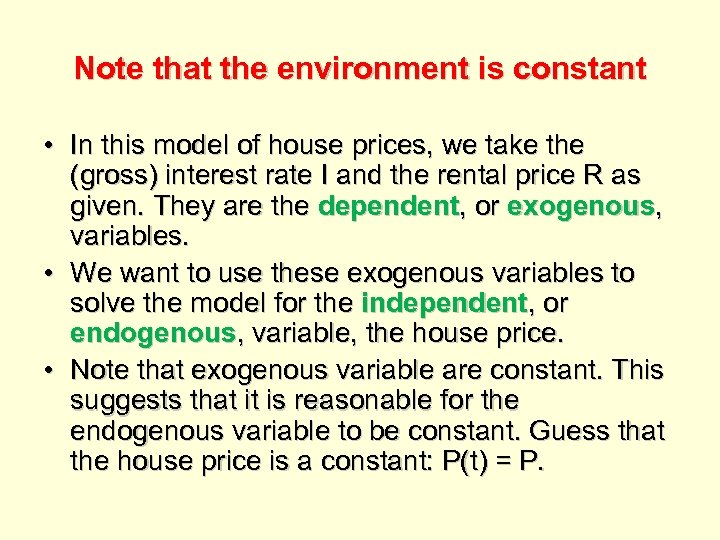Note that the environment is constant • In this model of house prices, we take the (gross) interest rate I and the rental price R as given. They are the dependent, or exogenous, variables. • We want to use these exogenous variables to solve the model for the independent, or endogenous, variable, the house price. • Note that exogenous variable are constant. This suggests that it is reasonable for the endogenous variable to be constant. Guess that the house price is a constant: P(t) = P.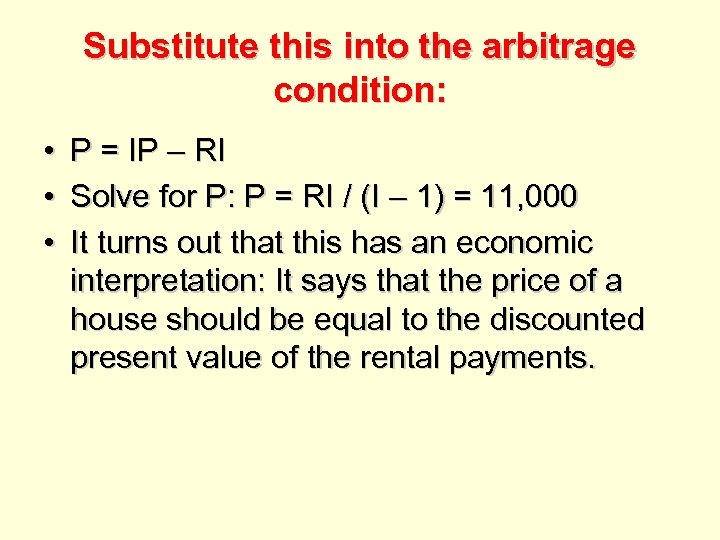Substitute this into the arbitrage condition: • • • P = IP – RI Solve for P: P = RI / (I – 1) = 11, 000 It turns out that this has an economic interpretation: It says that the price of a house should be equal to the discounted present value of the rental payments.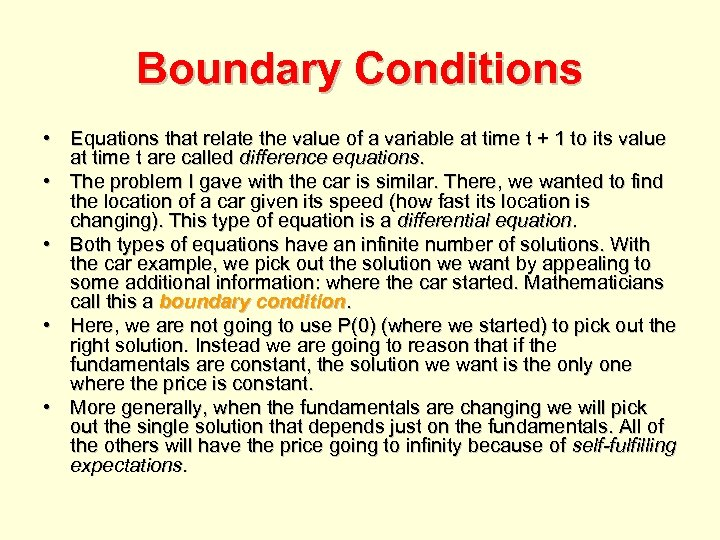Boundary Conditions • Equations that relate the value of a variable at time t + 1 to its value at time t are called difference equations. • The problem I gave with the car is similar. There, we wanted to find the location of a car given its speed (how fast its location is changing). This type of equation is a differential equation. • Both types of equations have an infinite number of solutions. With the car example, we pick out the solution we want by appealing to some additional information: where the car started. Mathematicians call this a boundary condition. • Here, we are not going to use P(0) (where we started) to pick out the right solution. Instead we are going to reason that if the fundamentals are constant, the solution we want is the only one where the price is constant. • More generally, when the fundamentals are changing we will pick out the single solution that depends just on the fundamentals. All of the others will have the price going to infinity because of self-fulfilling expectations.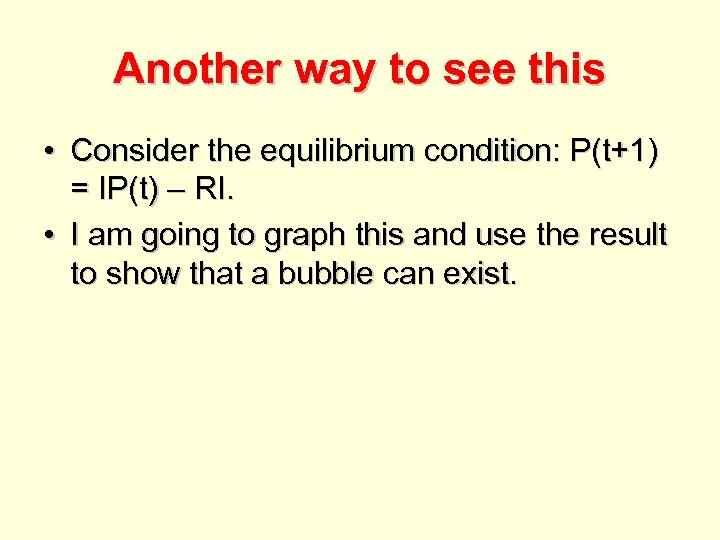Another way to see this • Consider the equilibrium condition: P(t+1) = IP(t) – RI. • I am going to graph this and use the result to show that a bubble can exist.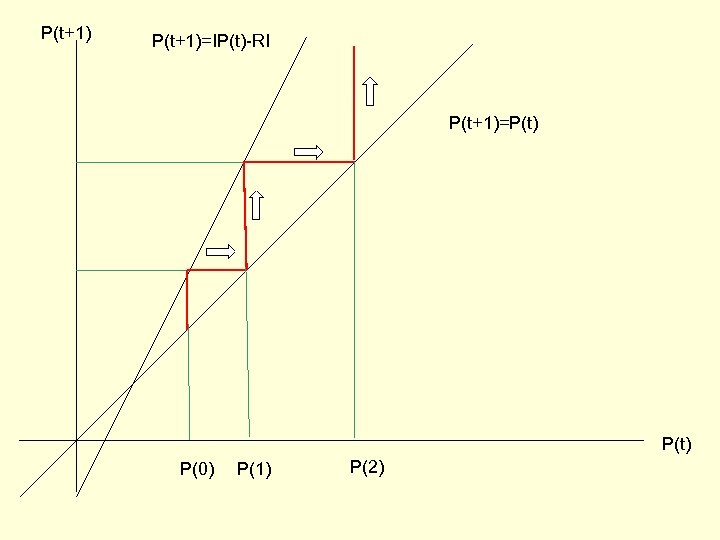P(t+1)=IP(t)-RI P(t+1)=P(t) P(0) P(1) P(2)This is related to coordination games • Suppose that two players are confronted with four tiles • They simultaneously select a tile. • If they select the same tile, they each get £ 1, 000. Otherwise, they get nothing. Player 1 sits here Player 2 sits here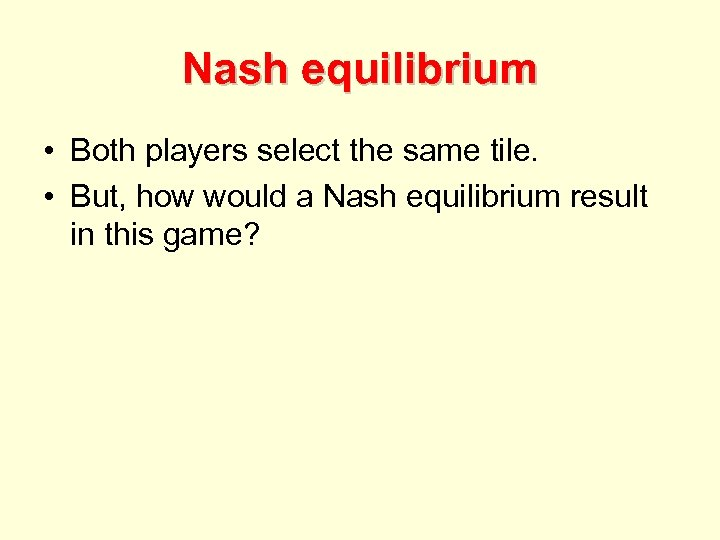Nash equilibrium • Both players select the same tile. • But, how would a Nash equilibrium result in this game?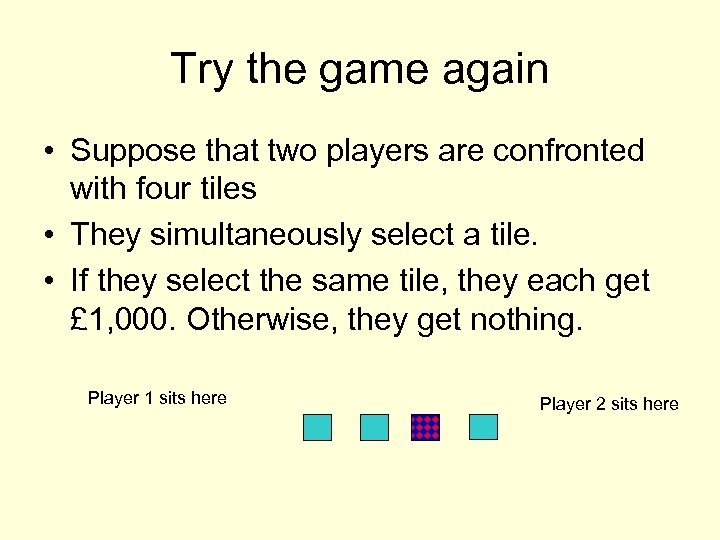Try the game again • Suppose that two players are confronted with four tiles • They simultaneously select a tile. • If they select the same tile, they each get £ 1, 000. Otherwise, they get nothing. Player 1 sits here Player 2 sits here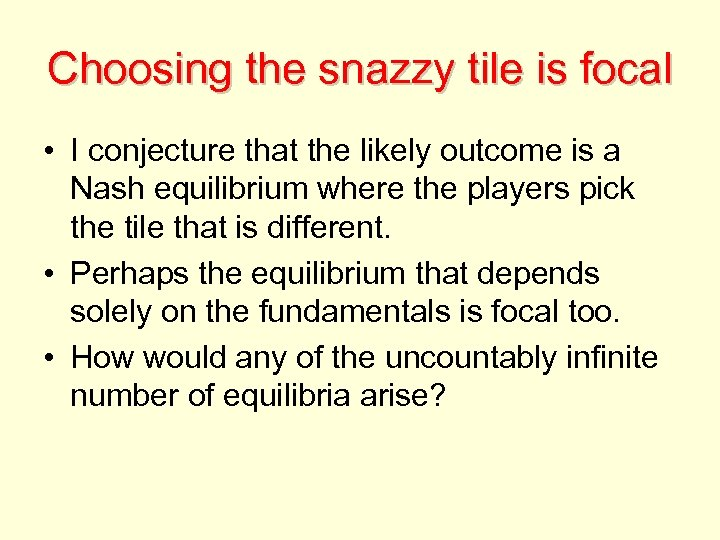Choosing the snazzy tile is focal • I conjecture that the likely outcome is a Nash equilibrium where the players pick the tile that is different. • Perhaps the equilibrium that depends solely on the fundamentals is focal too. • How would any of the uncountably infinite number of equilibria arise?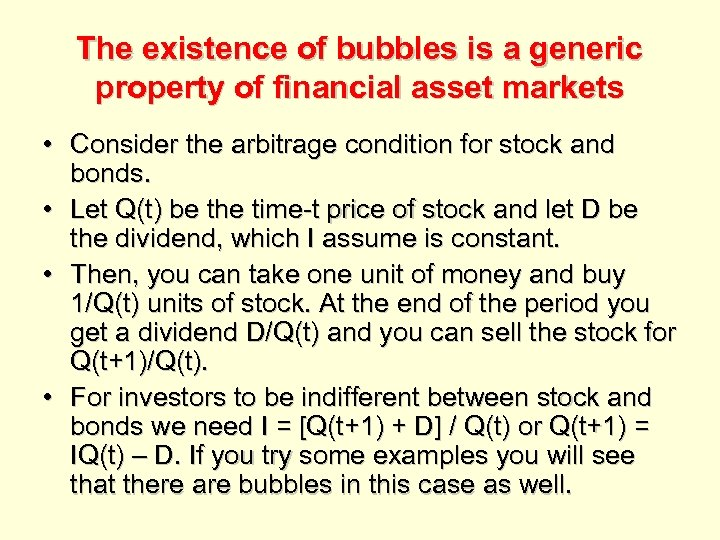The existence of bubbles is a generic property of financial asset markets • Consider the arbitrage condition for stock and bonds. • Let Q(t) be the time-t price of stock and let D be the dividend, which I assume is constant. • Then, you can take one unit of money and buy 1/Q(t) units of stock. At the end of the period you get a dividend D/Q(t) and you can sell the stock for Q(t+1)/Q(t). • For investors to be indifferent between stock and bonds we need I = [Q(t+1) + D] / Q(t) or Q(t+1) = IQ(t) – D. If you try some examples you will see that there are bubbles in this case as well.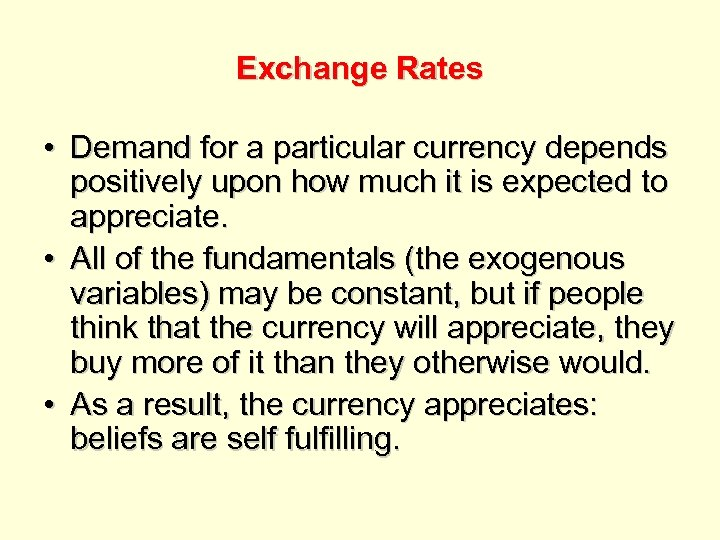Exchange Rates • Demand for a particular currency depends positively upon how much it is expected to appreciate. • All of the fundamentals (the exogenous variables) may be constant, but if people think that the currency will appreciate, they buy more of it than they otherwise would. • As a result, the currency appreciates: beliefs are self fulfilling.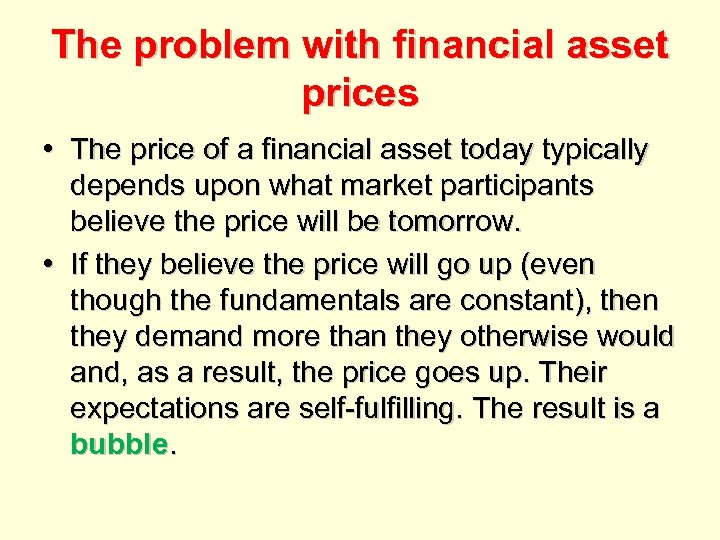The problem with financial asset prices • The price of a financial asset today typically depends upon what market participants believe the price will be tomorrow. • If they believe the price will go up (even though the fundamentals are constant), then they demand more than they otherwise would and, as a result, the price goes up. Their expectations are self-fulfilling. The result is a bubble.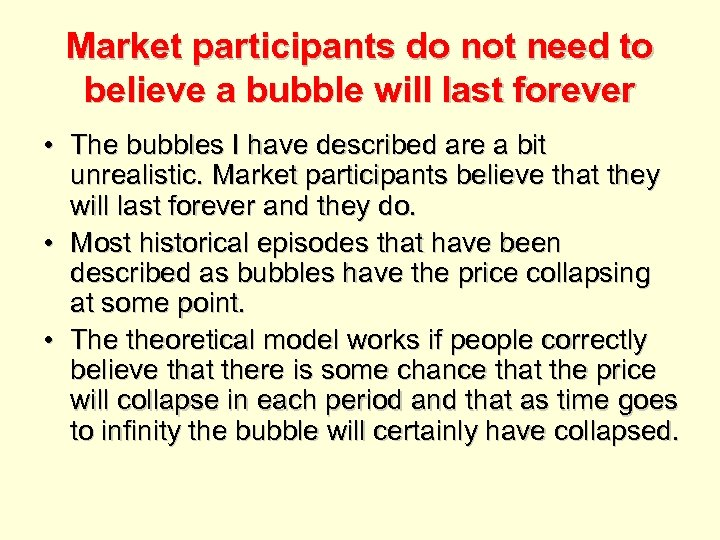Market participants do not need to believe a bubble will last forever • The bubbles I have described are a bit unrealistic. Market participants believe that they will last forever and they do. • Most historical episodes that have been described as bubbles have the price collapsing at some point. • The theoretical model works if people correctly believe that there is some chance that the price will collapse in each period and that as time goes to infinity the bubble will certainly have collapsed.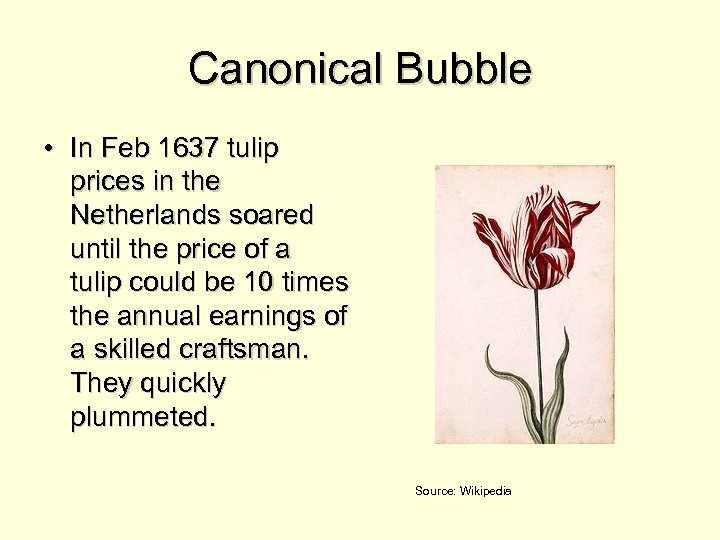Canonical Bubble • In Feb 1637 tulip prices in the Netherlands soared until the price of a tulip could be 10 times the annual earnings of a skilled craftsman. They quickly plummeted. Source: Wikipedia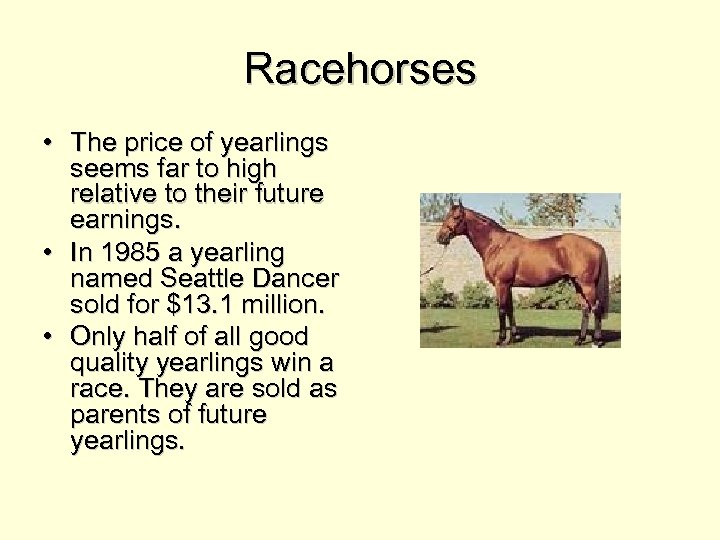Racehorses • The price of yearlings seems far to high relative to their future earnings. • In 1985 a yearling named Seattle Dancer sold for \$13. 1 million. • Only half of all good quality yearlings win a race. They are sold as parents of future yearlings.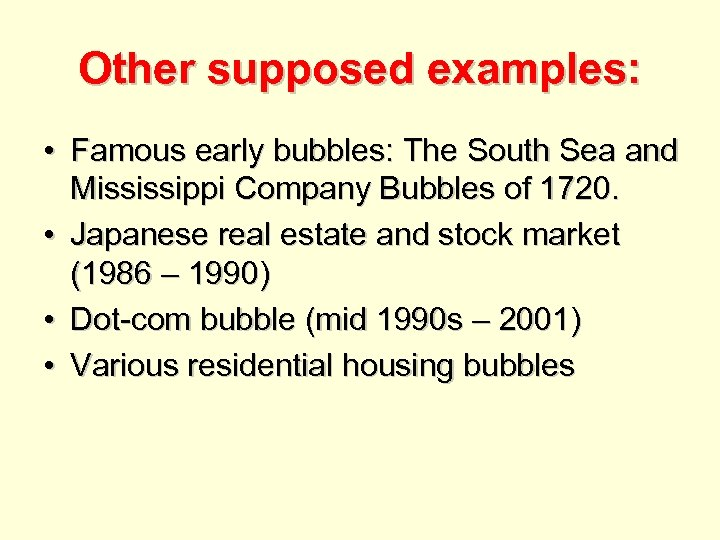Other supposed examples: • Famous early bubbles: The South Sea and Mississippi Company Bubbles of 1720. • Japanese real estate and stock market (1986 – 1990) • Dot-com bubble (mid 1990 s – 2001) • Various residential housing bubbles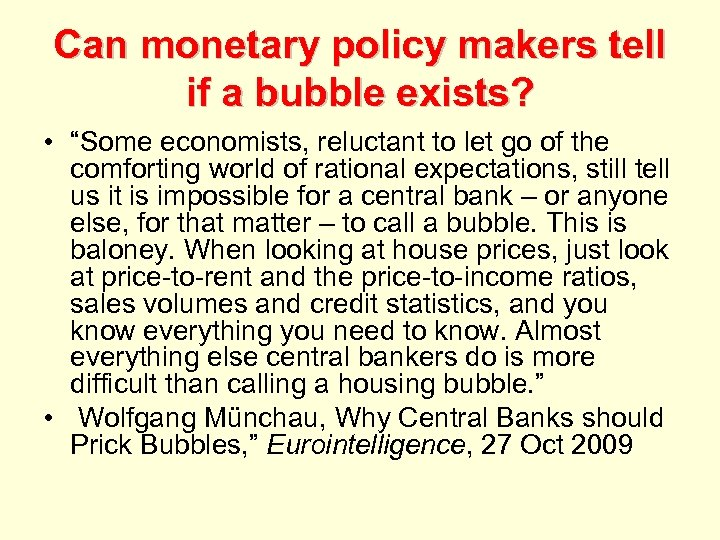Can monetary policy makers tell if a bubble exists? • “Some economists, reluctant to let go of the comforting world of rational expectations, still tell us it is impossible for a central bank – or anyone else, for that matter – to call a bubble. This is baloney. When looking at house prices, just look at price-to-rent and the price-to-income ratios, sales volumes and credit statistics, and you know everything you need to know. Almost everything else central bankers do is more difficult than calling a housing bubble. ” • Wolfgang Münchau, Why Central Banks should Prick Bubbles, ” Eurointelligence, 27 Oct 2009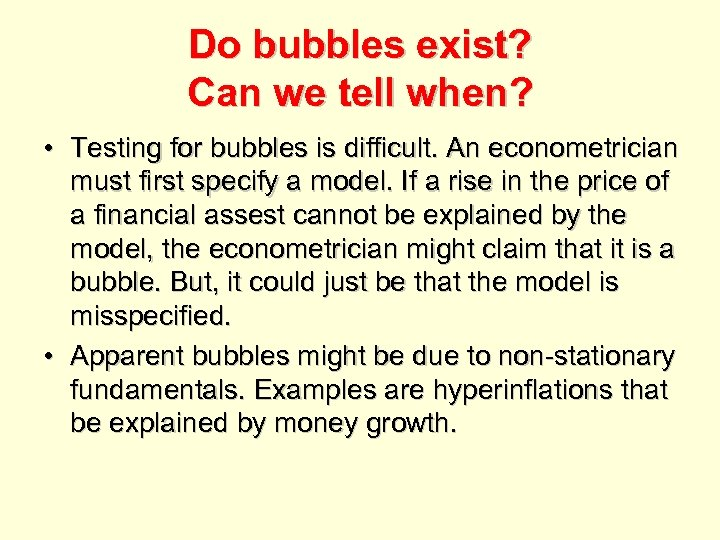Do bubbles exist? Can we tell when? • Testing for bubbles is difficult. An econometrician must first specify a model. If a rise in the price of a financial assest cannot be explained by the model, the econometrician might claim that it is a bubble. But, it could just be that the model is misspecified. • Apparent bubbles might be due to non-stationary fundamentals. Examples are hyperinflations that be explained by money growth.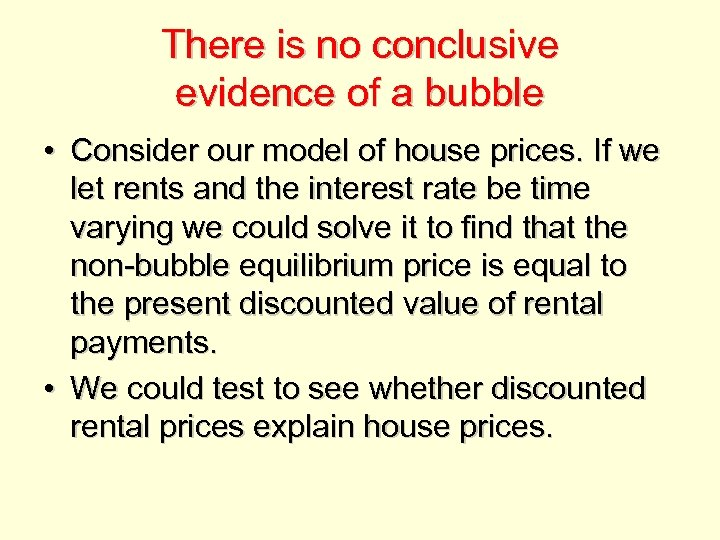There is no conclusive evidence of a bubble • Consider our model of house prices. If we let rents and the interest rate be time varying we could solve it to find that the non-bubble equilibrium price is equal to the present discounted value of rental payments. • We could test to see whether discounted rental prices explain house prices.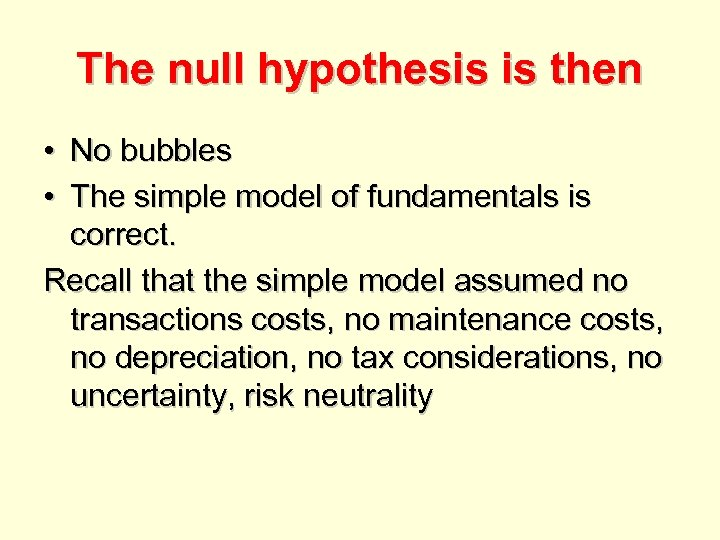The null hypothesis is then • No bubbles • The simple model of fundamentals is correct. Recall that the simple model assumed no transactions costs, no maintenance costs, no depreciation, no tax considerations, no uncertainty, risk neutrality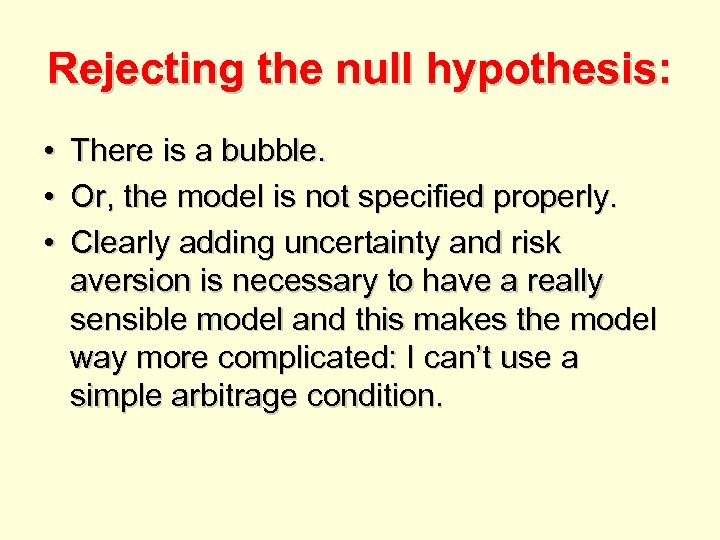Rejecting the null hypothesis: • • • There is a bubble. Or, the model is not specified properly. Clearly adding uncertainty and risk aversion is necessary to have a really sensible model and this makes the model way more complicated: I can’t use a simple arbitrage condition.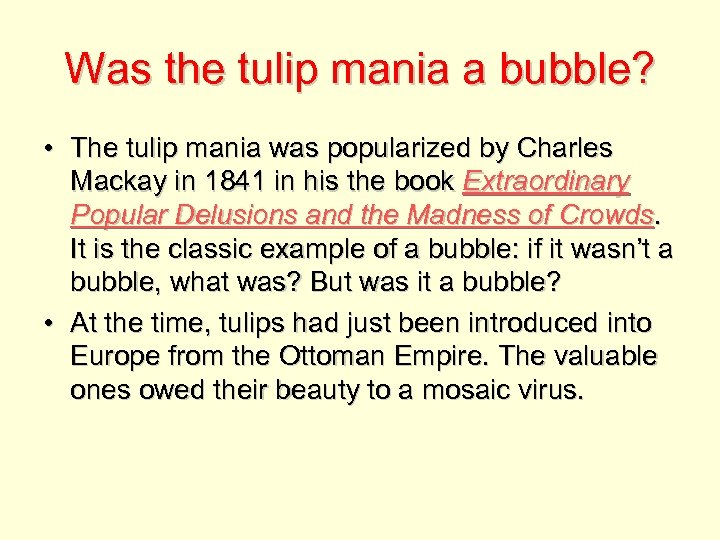Was the tulip mania a bubble? • The tulip mania was popularized by Charles Mackay in 1841 in his the book Extraordinary Popular Delusions and the Madness of Crowds. It is the classic example of a bubble: if it wasn’t a bubble, what was? But was it a bubble? • At the time, tulips had just been introduced into Europe from the Ottoman Empire. The valuable ones owed their beauty to a mosaic virus.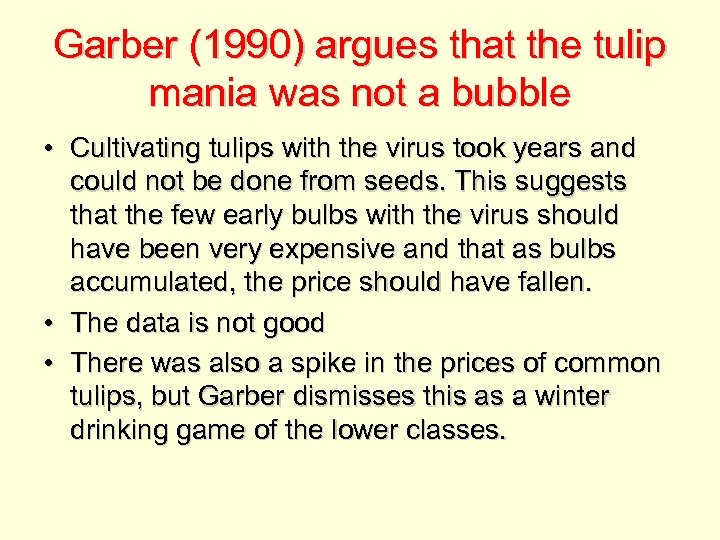Garber (1990) argues that the tulip mania was not a bubble • Cultivating tulips with the virus took years and could not be done from seeds. This suggests that the few early bulbs with the virus should have been very expensive and that as bulbs accumulated, the price should have fallen. • The data is not good • There was also a spike in the prices of common tulips, but Garber dismisses this as a winter drinking game of the lower classes.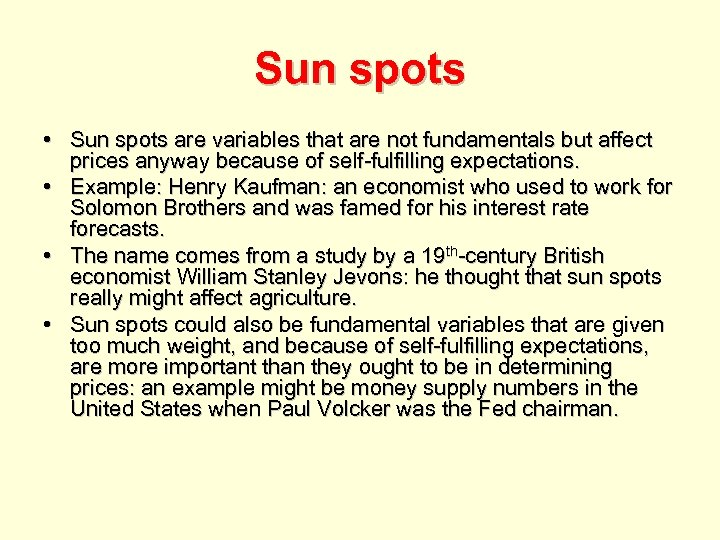Sun spots • Sun spots are variables that are not fundamentals but affect prices anyway because of self-fulfilling expectations. • Example: Henry Kaufman: an economist who used to work for Solomon Brothers and was famed for his interest rate forecasts. • The name comes from a study by a 19 th-century British economist William Stanley Jevons: he thought that sun spots really might affect agriculture. • Sun spots could also be fundamental variables that are given too much weight, and because of self-fulfilling expectations, are more important than they ought to be in determining prices: an example might be money supply numbers in the United States when Paul Volcker was the Fed chairman.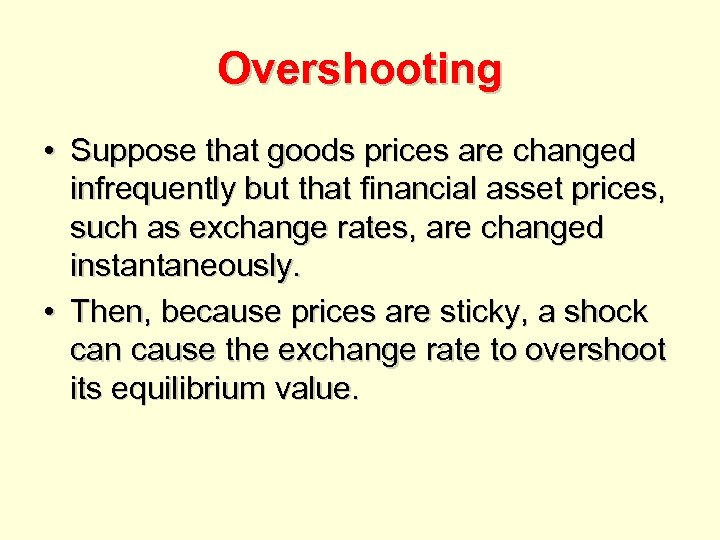Overshooting • Suppose that goods prices are changed infrequently but that financial asset prices, such as exchange rates, are changed instantaneously. • Then, because prices are sticky, a shock can cause the exchange rate to overshoot its equilibrium value.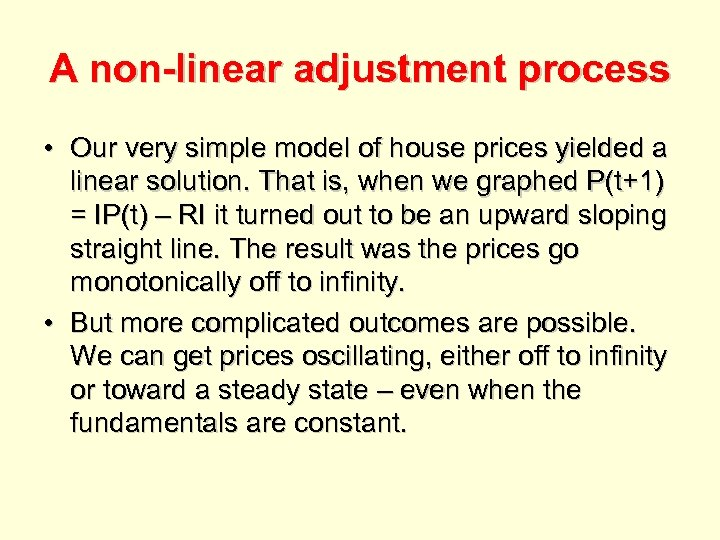A non-linear adjustment process • Our very simple model of house prices yielded a linear solution. That is, when we graphed P(t+1) = IP(t) – RI it turned out to be an upward sloping straight line. The result was the prices go monotonically off to infinity. • But more complicated outcomes are possible. We can get prices oscillating, either off to infinity or toward a steady state – even when the fundamentals are constant.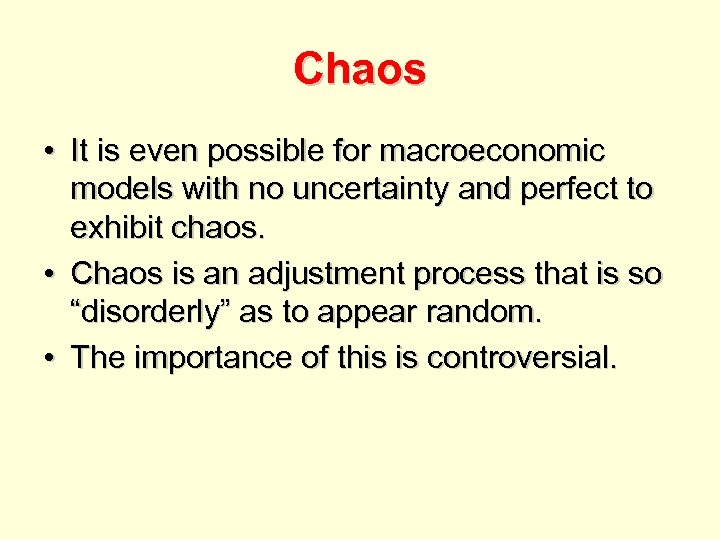Chaos • It is even possible for macroeconomic models with no uncertainty and perfect to exhibit chaos. • Chaos is an adjustment process that is so “disorderly” as to appear random. • The importance of this is controversial.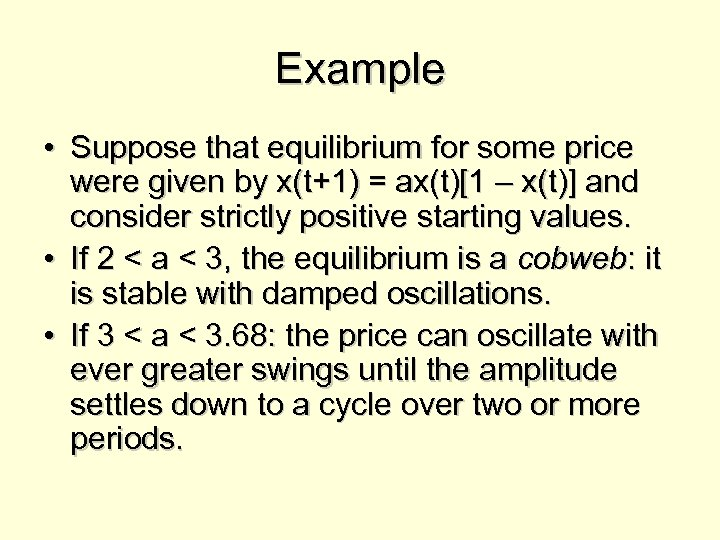Example • Suppose that equilibrium for some price were given by x(t+1) = ax(t)[1 – x(t)] and consider strictly positive starting values. • If 2 < a < 3, the equilibrium is a cobweb: it is stable with damped oscillations. • If 3 < a < 3. 68: the price can oscillate with ever greater swings until the amplitude settles down to a cycle over two or more periods.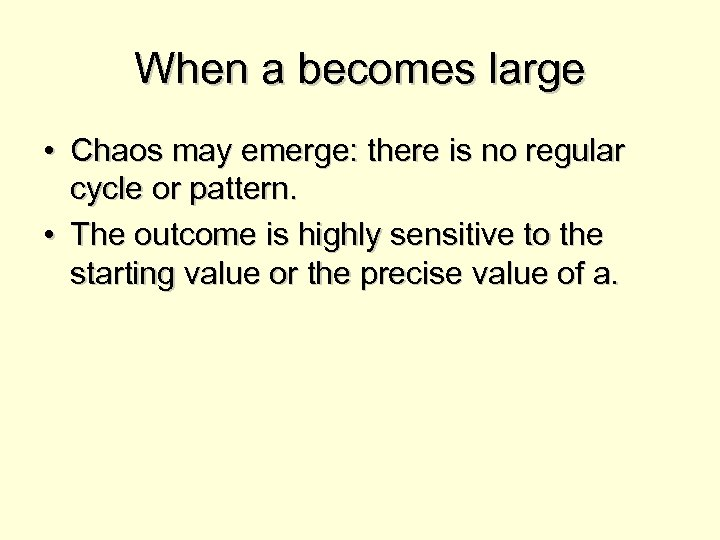When a becomes large • Chaos may emerge: there is no regular cycle or pattern. • The outcome is highly sensitive to the starting value or the precise value of a.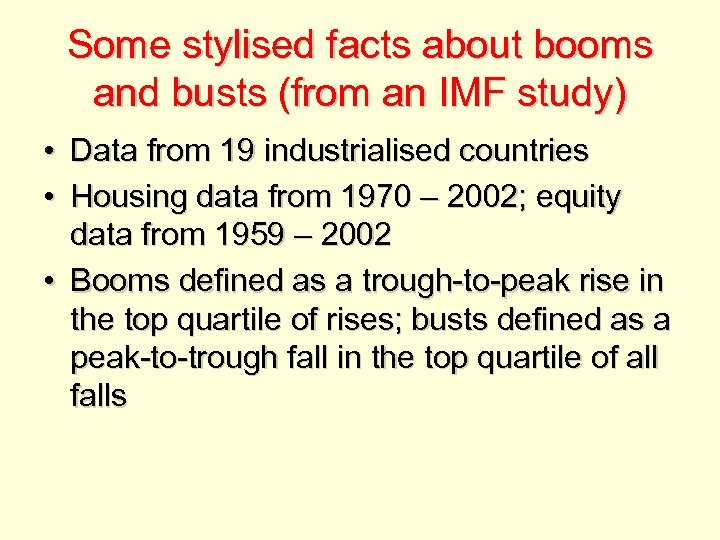Some stylised facts about booms and busts (from an IMF study) • Data from 19 industrialised countries • Housing data from 1970 – 2002; equity data from 1959 – 2002 • Booms defined as a trough-to-peak rise in the top quartile of rises; busts defined as a peak-to-trough fall in the top quartile of all falls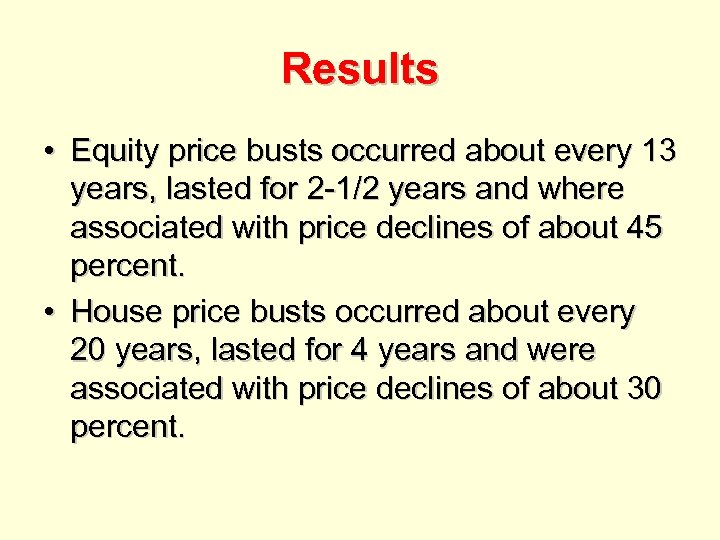Results • Equity price busts occurred about every 13 years, lasted for 2 -1/2 years and where associated with price declines of about 45 percent. • House price busts occurred about every 20 years, lasted for 4 years and were associated with price declines of about 30 percent.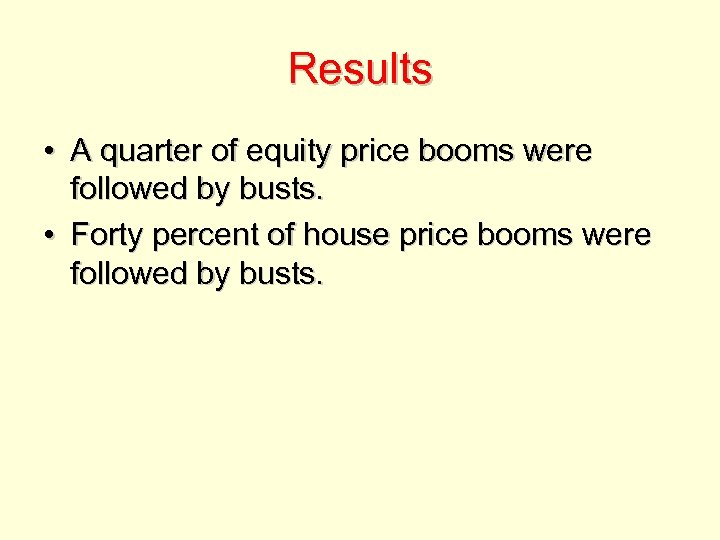Results • A quarter of equity price booms were followed by busts. • Forty percent of house price booms were followed by busts.